# (7)(20 points) (a) State the Fundamental Theorem of Finitely Generated Abelian Groups. (b) List all abelian...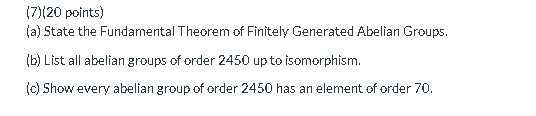(7)(20 points) (a) State the Fundamental Theorem of Finitely Generated Abelian Groups. (b) List all abelian groups of order 2450 up to isomorphism. (c) Show every abelian group of order 2450 has an element of order 70.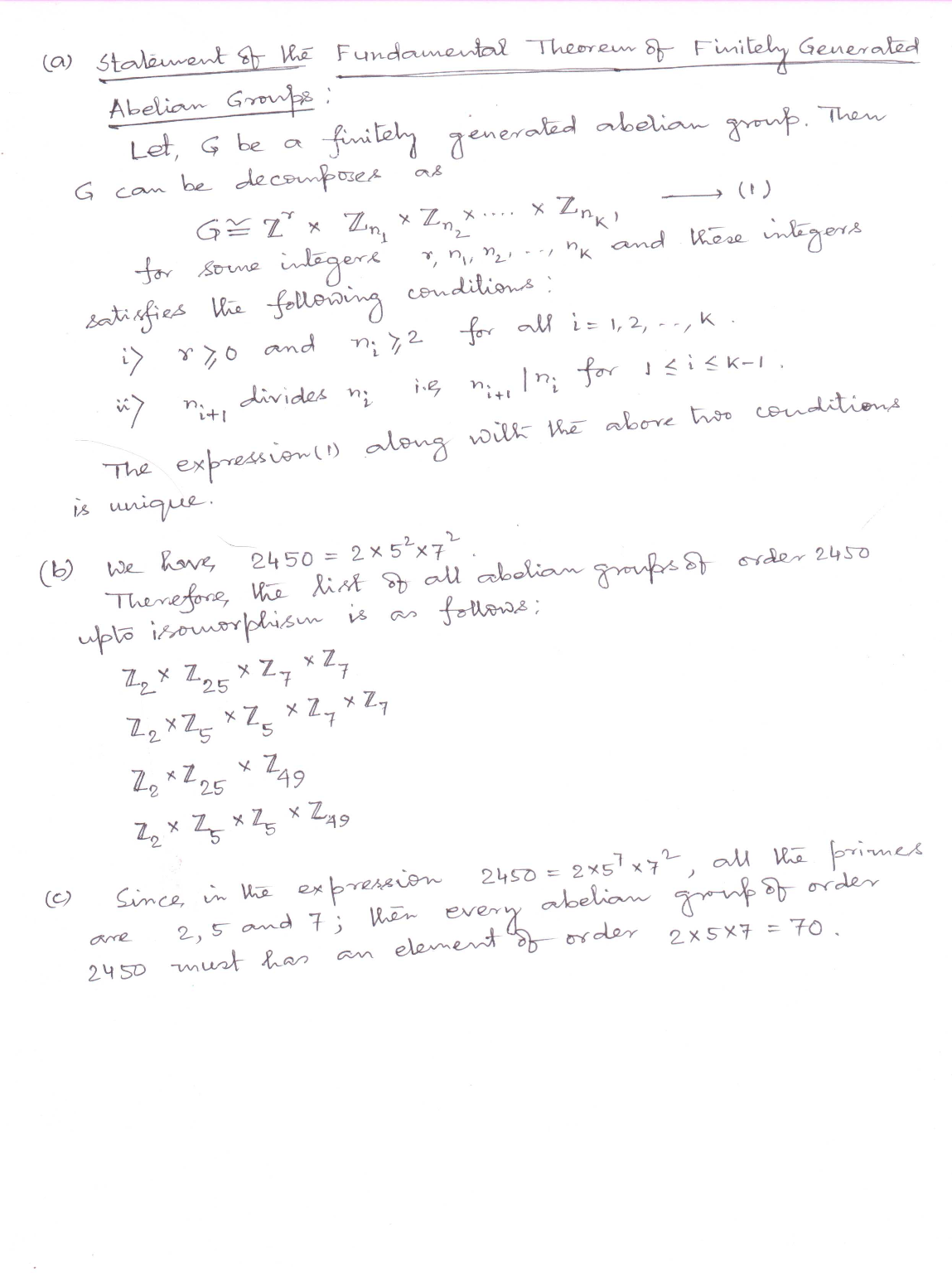##### Add Answer of: (7)(20 points) (a) State the Fundamental Theorem of Finitely Generated Abelian Groups. (b) List all abelian...
Similar Homework Help Questions
• ### (7)(20 points) (a) State the Fundamental Theorem of Finitely Generated Abelian Groups. (b) List all abelian...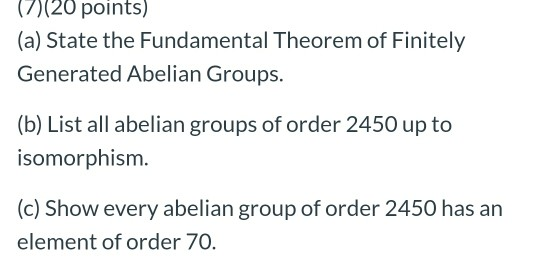(7)(20 points) (a) State the Fundamental Theorem of Finitely Generated Abelian Groups. (b) List all abelian groups of order 2450 up to isomorphism. (c) Show every abelian group of order 2450 has an element of order 70.

• ### (a) State the Fundamental Theorem of Finitely Generated Abelian Groups. (b) List all abelian groups of...

(a) State the Fundamental Theorem of Finitely Generated Abelian Groups. (b) List all abelian groups of order 2450 up to isomorphism. (c) Show every abelian group of order 2450 has an element of order 70.

• ### (7)(20 points) (a) State the Fundamental Theorem of Finitely Generated Abelian Groups. (b) List all abelian...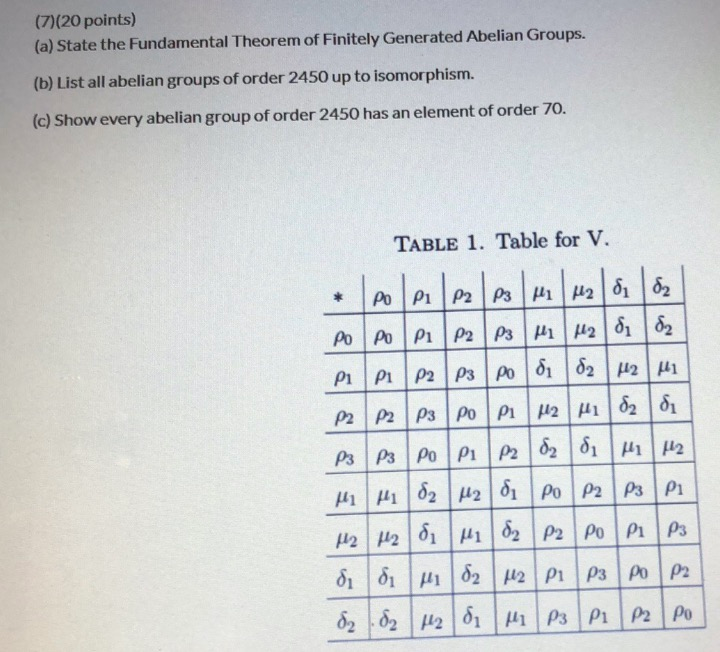(7)(20 points) (a) State the Fundamental Theorem of Finitely Generated Abelian Groups. (b) List all abelian groups of order 2450 up to isomorphism. (c) Show every abelian group of order 2450 has an element of order 70. TABLE 1. Table for V. * Po P1 P2 P3 Hi M28 d2 PoPoPIP2 P3MM 81 82 pi pi P2 P3 Podi 82 42 M P2|p2|p3|po|PI|HH|d2 di P3 P3 PoP1 P282 di Hi 112 M hd2 M2 di Po P2|p3|pl H2H2 di M82...

• ### need help ASAP with 6 and 7. Thank You! (6)(20 points) (a) Let G be a...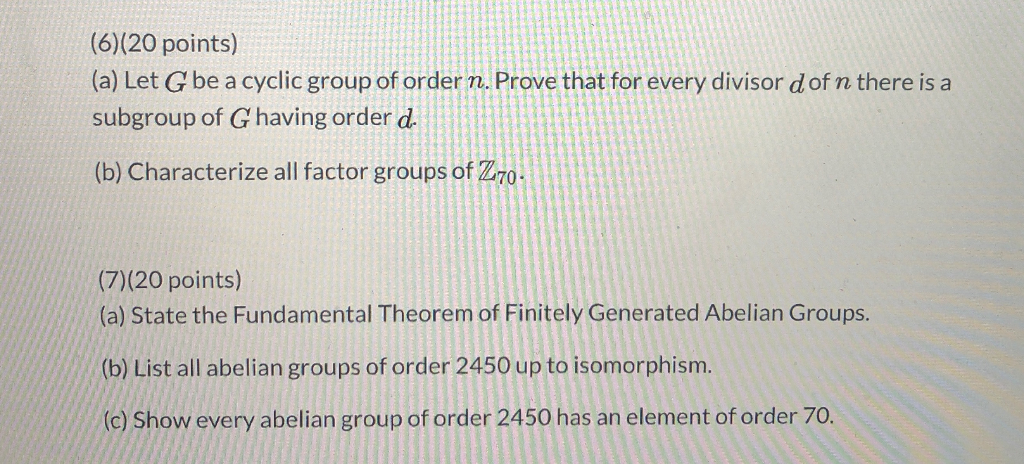need help ASAP with 6 and 7. Thank You! (6)(20 points) (a) Let G be a cyclic group of order n. Prove that for every divisor dof n there is a subgroup of Ghaving order d. (b) Characterize all factor groups of Z70. (7)(20 points) (a) State the Fundamental Theorem of Finitely Generated Abelian Groups. (b) List all abelian groups of order 2450 up to isomorphism. (c) Show every abelian group of order 2450 has an element of order 70.

• ### Use the Fundamental Theorem of Finitely Generated Abelian Groups to answer the following: a. Find all...

Use the Fundamental Theorem of Finitely Generated Abelian Groups to answer the following: a. Find all abelian groups, up to isomorphism, of order p3 where p is a prime b. Use part (a) with a suitable p to list all possible abelian groups that are isomorphic to (Z2x From this list, identify the abelian group that is isomorphic to (Z2xZ8)/(1, 4))

• ### (5)(20 points) In the problems below, give the order of the element in the indicated factor...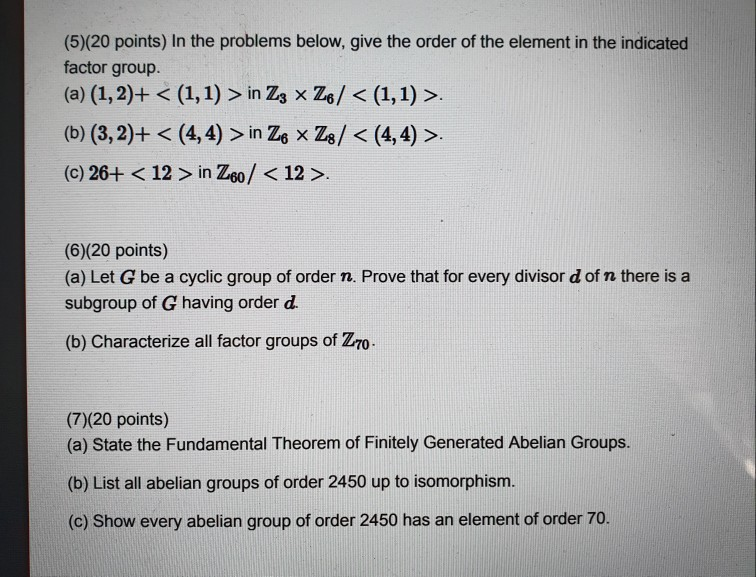(5)(20 points) In the problems below, give the order of the element in the indicated factor group. (a) (1, 2)+ < (1,1) > in Z3 x Z6/ < (1,1) >. (b) (3,2)+ < (4,4) > in Ze * Z8/ < (4,4) >. (c) 26+ < 12 > in Z60 / <12>. (6)(20 points) (a) Let G be a cyclic group of order n. Prove that for every divisor d of n there is a subgroup of G having order d....

• ### (1) (20 points) Using the Fundamental Theorem of Finitely Generated Abelian Groups, classify the following factor...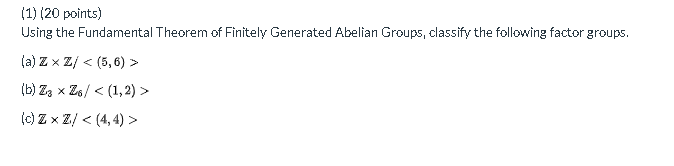(1) (20 points) Using the Fundamental Theorem of Finitely Generated Abelian Groups, classify the following factor groups. (a) ZxZ/ < (5,6) > (b) Zz Z6/ < (1,2) (0) ZxZ/ < (4,4) >

• ### ASAP (1) (20 points) Using the Fundamental Theorem of Finitely Generated Abelian Groups, classify the following...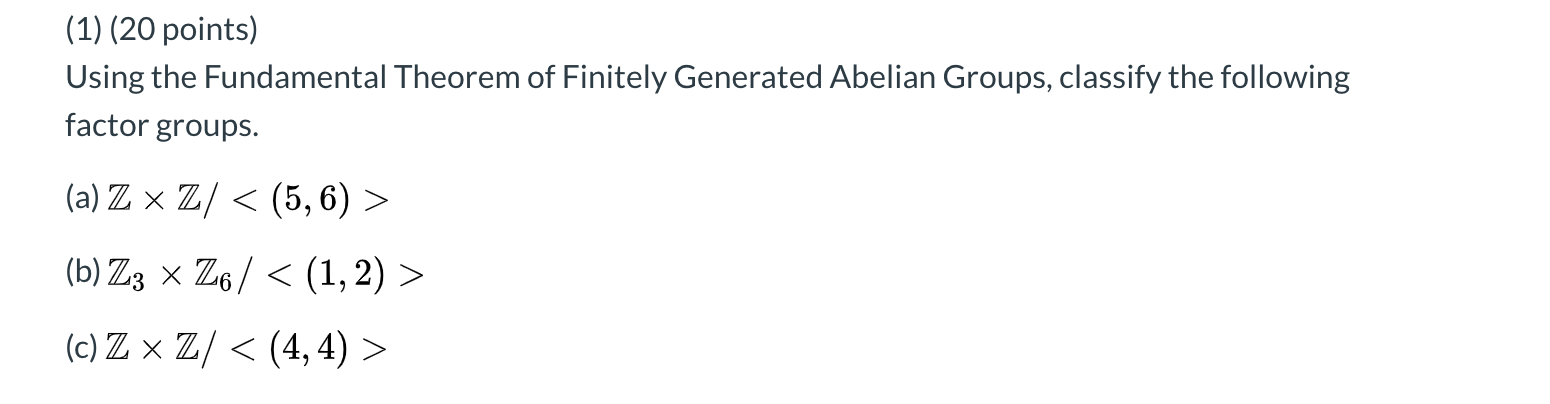ASAP (1) (20 points) Using the Fundamental Theorem of Finitely Generated Abelian Groups, classify the following factor groups. (a) Z x Z/ < (5,6) > (b) Z3 x Z6/ < (1, 2) > (c) Zx Z/ < (4,4) >

• ### (1) (20 points) Using the Fundamental Theorem of Finitely Generated Abelian Groups, classify the following factor...

(1) (20 points) Using the Fundamental Theorem of Finitely Generated Abelian Groups, classify the following factor groups. (a) Z × Z / < ( 5 , 6 ) > (b) Z 3 × Z 6 / < ( 1 , 2 ) > (c) Z x Z / < (4, 4) >

• ### Using the Fundamental Theorem of Finitely Generated Abelian Groups, classify the following factor groups. (a) Z...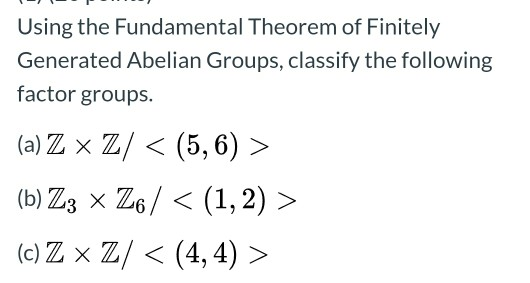Using the Fundamental Theorem of Finitely Generated Abelian Groups, classify the following factor groups. (a) Z x Z/ < (5,6) > (b) Z3 x Z6 / < (1,2) > (c) Z x Z/ < (4,4) >

Free Homework App